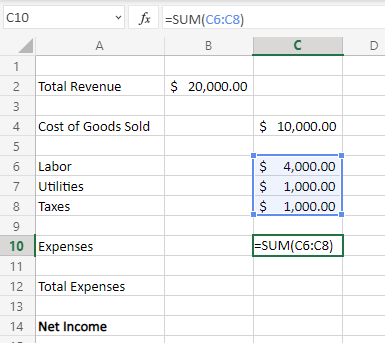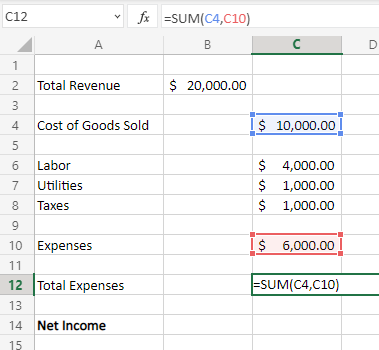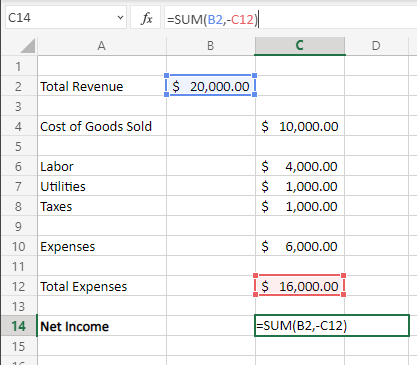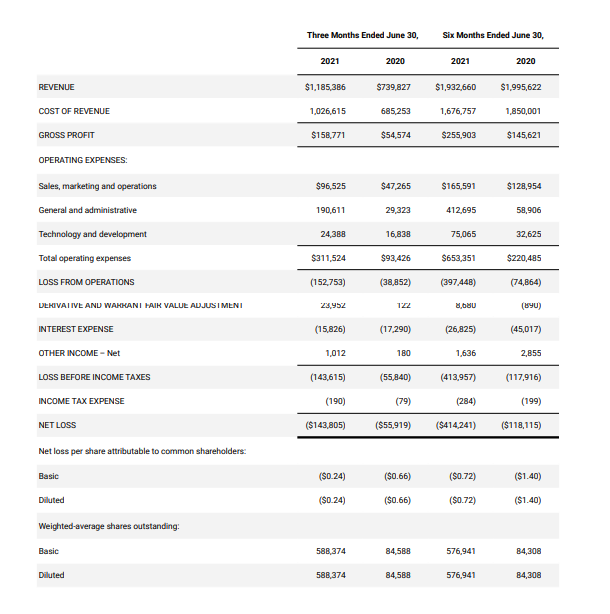# How to Calculate Net Income

Having a clear understanding of net income is critical to measuring how profitable a business is. The net income definition is the earnings which remain after expenses, interest, and applicable taxes are subtracted from revenue. Also known as ‘net earnings’ or ‘net profit’, net income is located at the bottom of the income statement, given that it is the total profit which remains after deducting expenses.

For business owners or investors determining how profitable a business is, calculating net income is an invaluable tool. If total expenses exceed total revenue, then a business will have a negative net income and therefore unprofitable. Business owners may need to cut costs to lower total expenses or try to increase sales to generate higher revenue to increase net income. This is why net income is crucial to identifying where a business needs to improve its efforts.

This article will illustrate the net income formula, how to calculate net income step by step, and provide examples on how to apply it in various scenarios.

## Net Income Definition

An indicator of the profitability of a business, net income is also referred to as the ‘bottom line’ of a business (coined this term because it is found near the bottom of the income statement). Net income is total revenue minus total expenses. Net income is one of the most important values on a company’s financial statements, as it is used to determine earnings per share.

## What is Net Income

### Formula

The net income formula is calculated by subtracting total revenue from the cost of goods sold and other expenses. For businesses which provide services instead of goods, you would replace cost of goods sold with any costs to provide the service. Expenses include operating expenses, interest, taxes, and other types of expenses. Net income is measured in units of dollars.

Net Income = Total Revenue – Cost of Goods Sold – Expenses

To simplify the formula further, cost of goods sold and expenses can be combined into ‘total expenses.’ Net income can be either positive or negative, depending on a business’ profitability.

Net Income = Total Revenue – Total Expenses

## How to Calculate Net Income

### Calculate Step by Step

1. Add up all expenses incurred over a time period (i.e., taxes, depreciation, or other expenses)
2. Add cost of goods sold and expenses to determine total expenses
3. Subtract total revenue from total expenses

### Example

A furniture store needs to determine its net income for the second quarter of 2020.

Based on their operations, the business calculated the following values:

Total Revenue: \$200,000

Cost of Goods Sold: \$100,000

Labor: \$50,000

Utilities: \$3,000

Rent: \$20,000

Marketing: \$2,000

Taxes: \$10,000

First, the business owner will add all of the expenses for the period:

Expenses = Labor + Utilities + Rent + Marketing + Taxes

Expenses = \$50,000 + \$3,000 + \$20,000 + \$2,000 + \$10,000

Expenses = \$85,000

Next, the business owner will add the cost of goods sold to these expenses to calculate total expenses:

Total Expenses = cost of goods sold + expenses

Total Expenses = \$100,000 + \$85,000

Total Expenses = \$185,000

Now, the business owner will subtract total revenue from total expenses to determine net income:

Net Income = Total Revenue – Total Expenses

Net Income = \$200,000 – \$185,000

Net Income = \$15,000

Here we can see that the furniture store was profitable over the second quarter of 2020, with a net income of \$15,000.

## Tips for Calculating Net Income

### Tip Number 1:

If a company provides multiple types of products, make sure the revenue produced from all products is reflected in the total revenue. Similarly, make sure the cost of goods sold from all products is reflected in the total expenses. All products must be considered, since net income is a measure of all operations of a business over a given time period.

### Tip Number 2:

A variety of different costs add up to the total expenses over a time period. It is important to represent these costs when solving for net income. Make sure to add values such as taxes, interest on debt, operating expenses, overhead costs, utilities, advertising, and especially labor (also known as payroll). If any of these costs are not represented in the value of total expenses, the true profitability of a business could be misrepresented.

### Tip Number 3:

Net income can also be solved by subtracting gross income from expenses. Gross income is total revenue minus the cost of goods sold. Expenses are then subtracted from gross income to solve for net income. Knowing this trick can be helpful if you are ever in a situation where you only have a value for gross income.

## Calculating Net Income In Excel

Microsoft Excel can be used to effectively calculate net income. Since most of the information used to solve for net income is found in a company’s financial statements, these statements are commonly converted into Excel files for quick calculations. When setting up an annual net income calculator, follow these simple steps.

For this example, we will calculate the net income of a pottery store for the first quarter of 2021.We can see that during the quarter, the pottery store generated a total revenue of \$20,000, along with a cost of goods sold of \$10,000. Expenses for the period included the cost of labor, utilities, and taxes.

To calculate the expenses for the quarter, we must add labor, utilities, and taxes by inserting the following formula into the expenses cell of C10:

=SUM(C6:C8)Once expenses have been added, they must now be added to costs of goods sold in order to solve for total expenses. To calculate total expenses, insert the following formula into the total expenses cell of C12:

=SUM(C4,C10)Now that we have calculated total expenses, the last step is to subtract total expenses from total revenue to calculate net income. To solve for net income, insert the following formula into the net income cell of C14 (make sure to put a “-“ in front of C12):

=SUM(B2,-C12)

In this case, the net income will end up being \$4,000. Remember when solving for the net income that the value can be negative, showing that a company was not profitable over the given time period.

## Where to Find Net Income: The Income Statement

When looking for the net income of a business, remember that net income is also commonly referred to as the business’ bottom line, since it is found at the bottom of the income statement. This will always remind you exactly where to find it.

Net income is the most important indicator of a company’s profitability. It can be used by business owners to determine if revenue must be increased or expenses must be lowered by cutting costs. It can also be used by investors to measure the success of a business.

An actual example of this is when a company releases quarterly results to the public, such as when Opendoor Technologies Inc. released second quarter results for 2021. As found on page 19 of the results:

Opendoor reports net income after subtracting total expenses. Including cost of revenue, sales, development, and interest expenses, from revenue. Since this resulted in a loss, Opendoor reports net income as ‘net loss’ in this scenario. This is a common occurrence among other publicly-traded companies when reporting a loss.## Takeaways

Net income is total revenue minus cost of goods sold and expenses (known as total expenses). Net income can be either positive or negative and is an important indicator of the profitability of a business. Make sure to consider all expenses to accurately calculate net income.

### Different Uses and Applications of organic chemistry

The uses and applications of organic chemistry range from life-saving pharmaceutical discoveries to the vibrant colors of fruits and vegetables we see daily. In this

### Ace organic chemistry- tips and tricks, cheat sheets, summary

Mastering organic chemistry takes time and practice. It might seem a daunting task at once if the right approach is not adopted. But in our

### Named reactions of organic chemistry- an overview

This article on organic reactions is a special one in our organic chemistry series. It will guide you through how chemical transformations unlock diversity at

### Organic Spectroscopy

Organic spectroscopy can be used to identify and investigate organic molecules. It deals with the interaction between electromagnetic radiation (EMR) and matter. These waves travel